# Definition--Equation Concepts--Addition Property of Equality

This is part of a collection of definitions on the topic of Equations. These downloadable images can easily be incorporated into a lesson.

### To see the complete collection of definitions on this topic, click on this link.

The next section provides background information on equations and properties you can use to make it easier to solve them.

## Equations: When Two Quantities Are Equal

An equation is like a scale that’s balanced. Take a look at this illustration.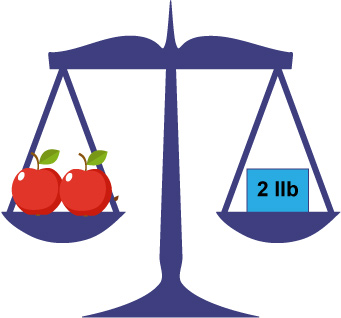The two apples on the left are balanced by the 2-pound weight on the right. The balanced scale is a type of equality statement.

We could also look at the balanced scaled this way.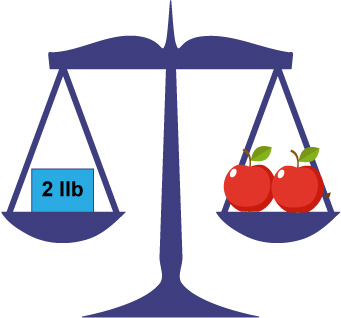This is the same equality statement as the previous one and is an example of the Symmetric Property of Equality.

## The Symmetric Property of Equality

Look at this equation.Because of the Symmetric Property of Equality, you can also write the equation this way.Take a look at this equation.The math convention is to have the variable on the left side of the equation. This is where the Symmetric Property of Equality comes in handy. We can rewrite the equation this way.In this form, we can now solve the equation.## Use the Symmetric Property of Equality to help you solve equations.Math Properties

As you work with numbers, it's important to know properties of operations and equations. Learn these and you'll have an easier time combining certain expressions.

Let's start by looking at the properties relating to addition.

Commutative Property.  When adding two numbers, it doesn't matter in what order you add them.  The Commutative Property of Addition means that for any two numbers a and b,

### a + b = b + a

Why is this an important property to use? Take a look at this expression:

### 7 + 25 + 3

Use the Commutative Property to rewrite the expression this way

### 7 + 3 + 25

Do you see how the 7 and 3 easily combine to form a 10, making the sum much easier to find:

### 7 + 3 + 25 = 10 + 25 = 35

Look for opportunities to use the Commutative Property to simplify addition.

Associative Property.  When adding three or more numbers, you can choose any pair of numbers to add first. The Associative Property of Addition means that you can add any pair of numbers first.

### (a + b) + c = a + (b + c)

Here's how to use the Associative Property to simplify addition:

### (25 + 3) + 7 = 25 + (3 + 7) = 25 + 10 = 35

In this case using the Associative Property simplifies the addition.

### Multiplication Properties

Commutative Property.  When multiplying two numbers, it doesn't matter in what order you multiply them.  The Commutative Property of Multiplication means that for any two numbers a and b,

### a • b = b • a

Why is this an important property to use? Take a look at this expression:

### 25 • 27 • 4

Use the Commutative Property to rewrite the expression this way

### 27 • 25 • 4

Do you see how the product of 25 and 4 is 100, making the product much easier to find:

### 27 • 25 • 4 = 27 • 100 = 2700

Look for opportunities to use the Commutative Property to simplify multiplication.

Associative Property.  When multiplying three or more numbers, you can choose any pair of numbers to multiply first. The Associative Property of Multiplication means that you can multiply any pair of numbers first.

### (a • b) • c = a • (b • c)

Here's how to use the Associative Property to simplify addition:

### (37 • 20) • 5 = 37 • (20 • 5) = 37 • 100 = 3700

In this case using the Associative Property simplifies the multiplication.

### Equation Properties

As you solve an equation, use the properties shown above in the solution process. Also, take advantage of the following equation properties

Addition Property of Equality.  When solving an equation, you may have to remove a number from one side of the equation in order to isolate the variable. Use the Addition Property of Equality, which states that whatever you add to one side of an equation, you also have to add to the other side of the equation.Here is an example of using the Addition Property of Equality to solve an equation: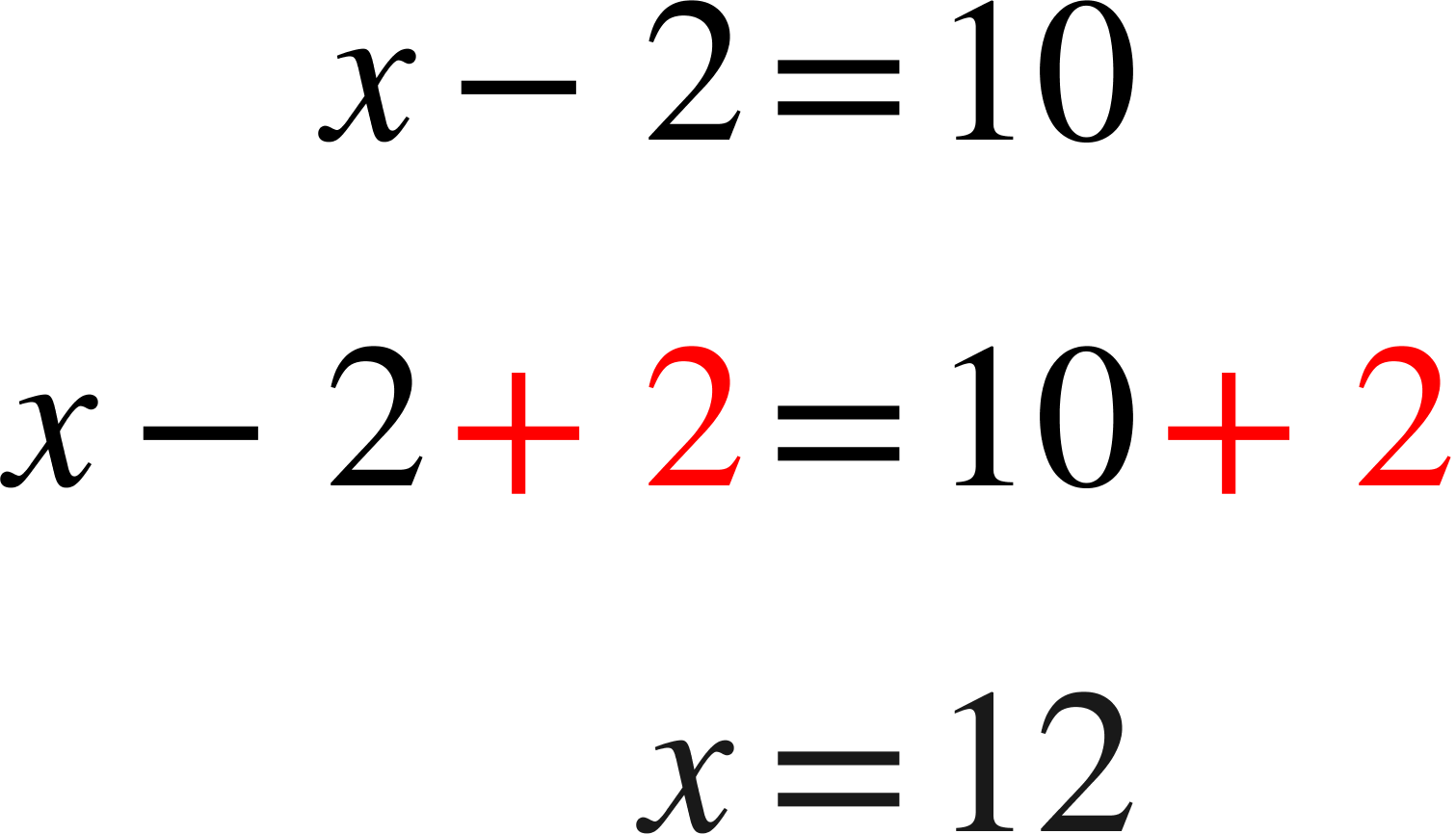Notice how 2 is added to both sides in order to get x by itself to solve the equation.

Subtraction Property of Equality.  When solving an equation, you may have to remove a number from one side of the equation in order to isolate the variable. Use the Subtraction Property of Equality, which states that whatever you subtract from one side of an equation, you also have to subtract from the other side of the equation.Here is an example of using the Subtraction Property of Equality to solve an equation: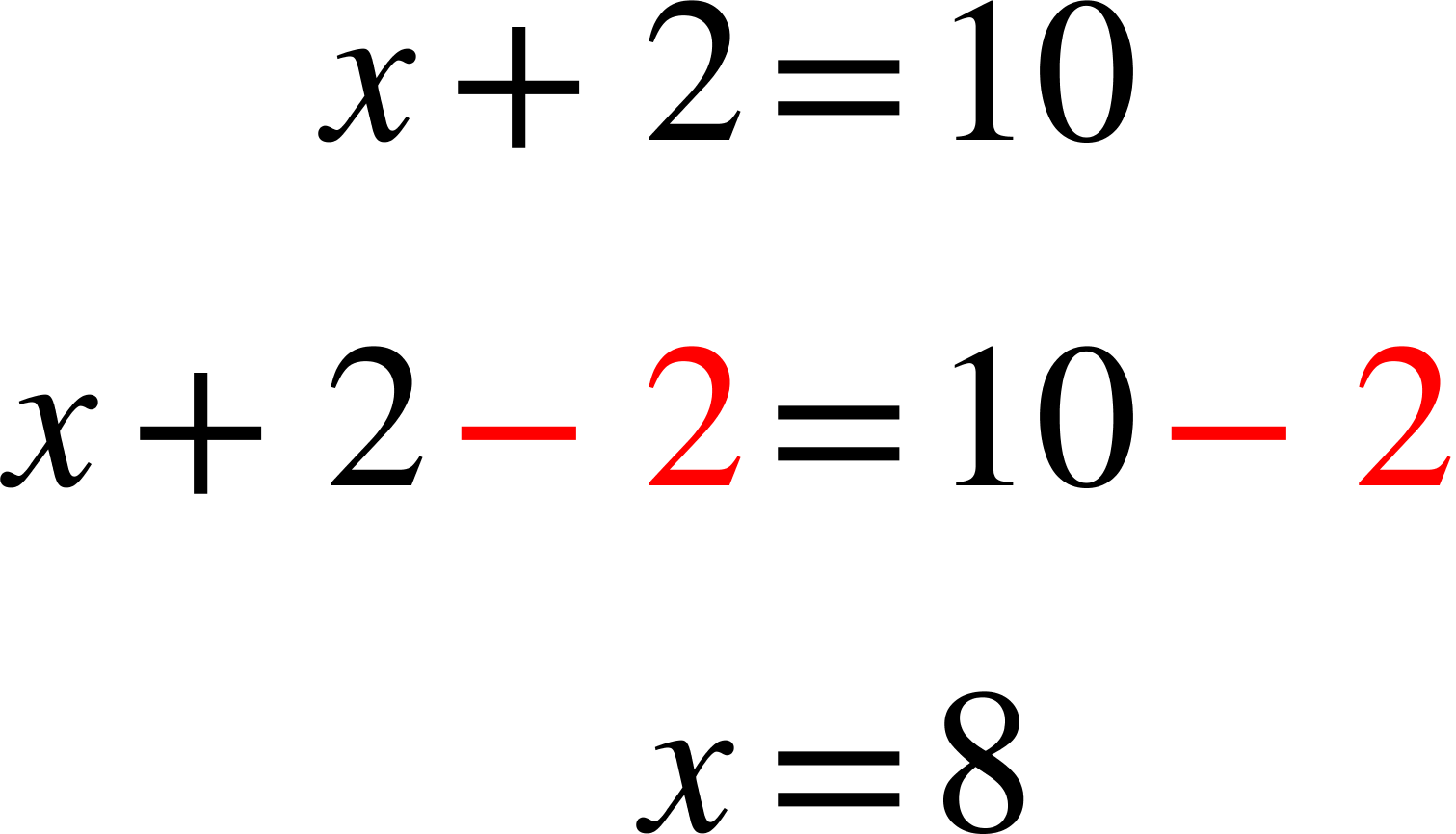Notice how 2 is subtracted from both sides in order to get x by itself to solve the equation.

Multiplication Property of Equality.  When solving an equation, you may have to remove a number from one side of the equation in order to isolate the variable. Use the Multiplication Property of Equality, which states that whatever you multiply by on one side of an equation, you also have to multiply by on the other side of the equation.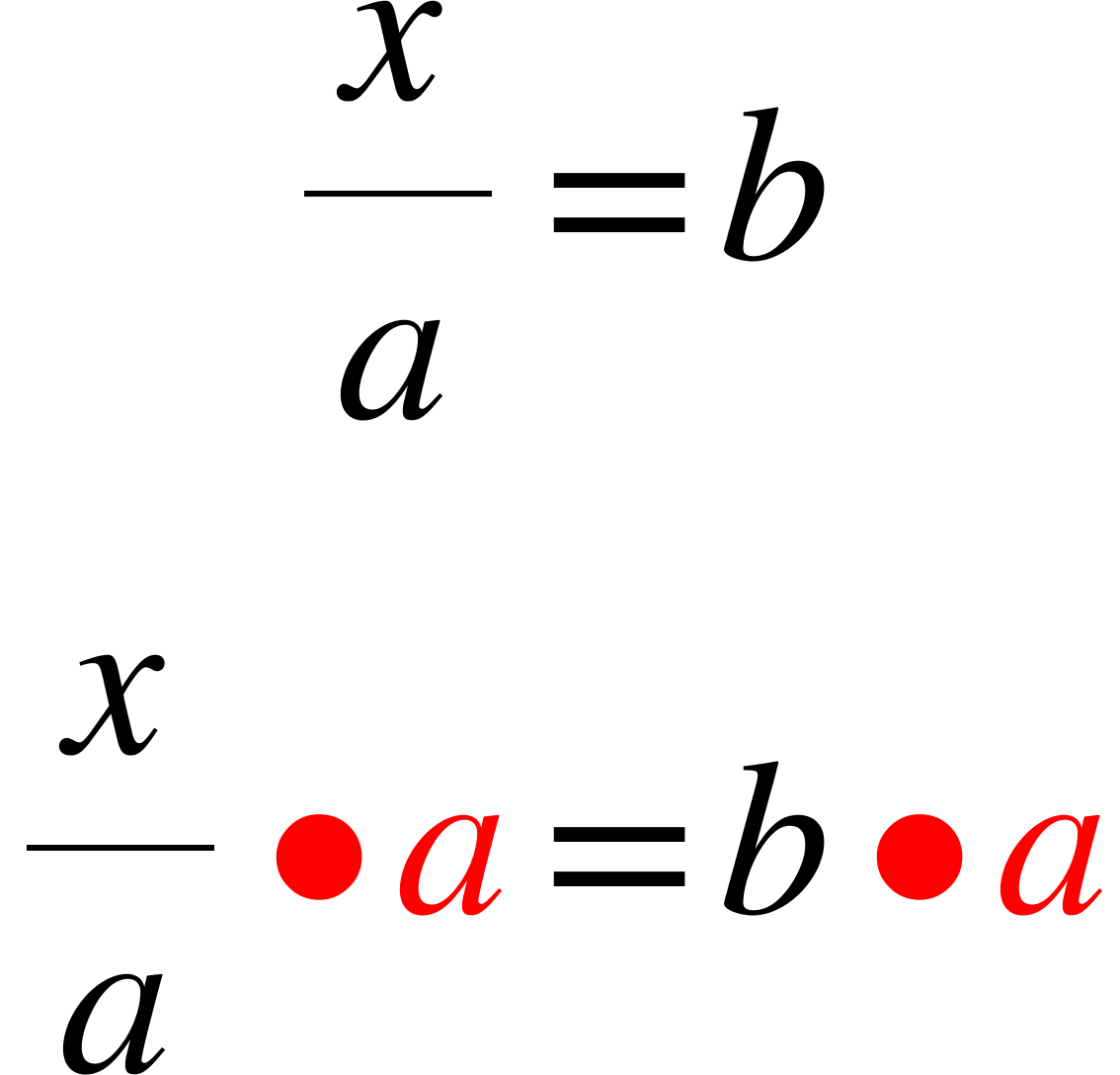Here is an example of using the Multiplication Property of Equality to solve an equation: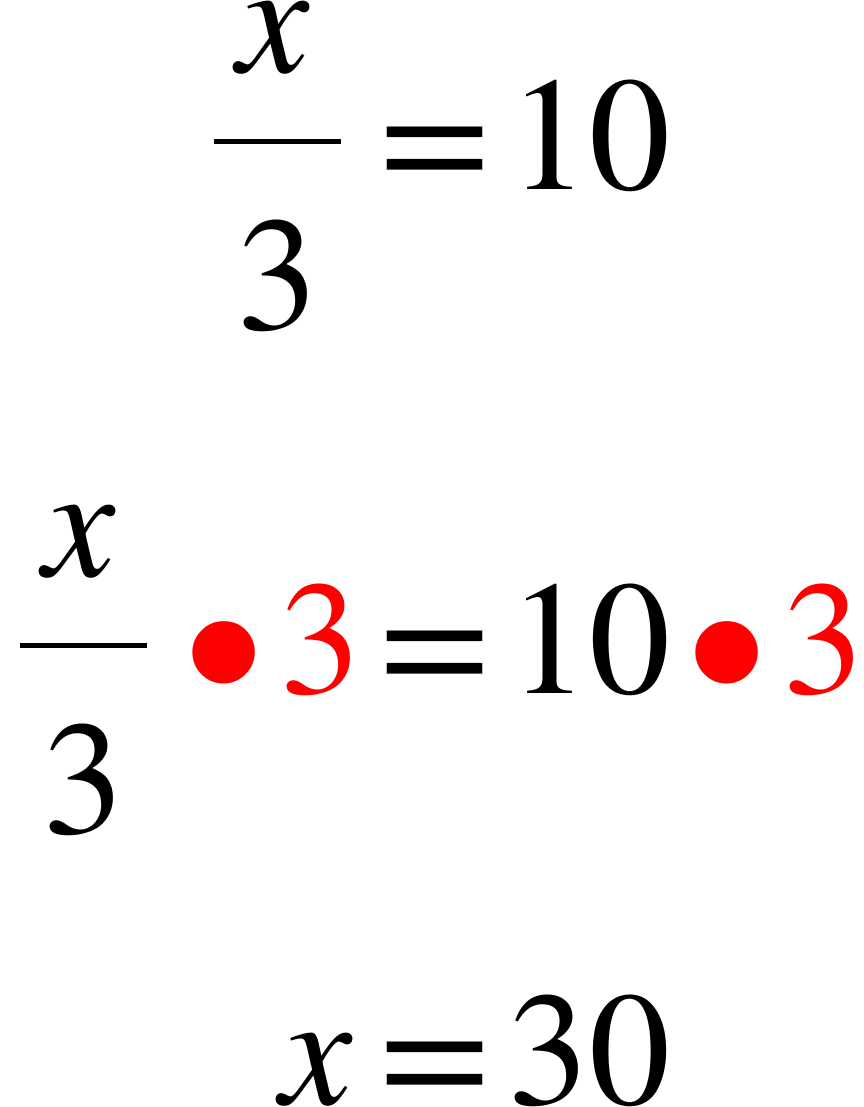Division Property of Equality.  When solving an equation, you may have to remove a number from one side of the equation in order to isolate the variable. Use the Division Property of Equality, which states that whatever you divide by on one side of an equation, you also have to divide by on the other side of the equation. Note: You cannot divide by zero.Here is an example of using the Division Property of Equality to solve an equation:Notice how both sides of the equation are divided by 2 in order to get x by itself to solve the equation.